## LetsPlayMaths.Com

WELCOME TO THE WORLD OF MATHEMATICS

# Class-7 Lines and Angles

Introduction to Lines and Angles

Complementary Angles

Supplementary Angles

Linear Pair

Angles About a Point

Vertically Opposite Angles

Angles on One Side of a Straight Line

Concept of Transversal

Interior Angles

Exterior Angles

Corresponding Angles

Alternate Interior Angles

Alternate Exterior Angles

Co-interior Angles

Properties of Angles Made by a Transversal with Two Parallel Lines

Lines and Angles Test

Lines and Angles Worksheet

## Introduction to Lines and Angles

In class 6, we have learnt about some basic concepts of geometry. Here, we are going to learn about complementary angles, supplementary angles, adjacent angles, sum of angles at a point, and many more concepts.

## Complementary Angles

Two angles are known as complementary angles if the sum of their measures is 90°. Each angle is called the complement of the other angle. Let's see some examples to understand it better.

Example 1. Prove that below provided angles are complementary angles.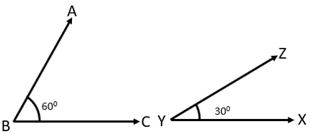Here, ∠ABC + ∠XYZ = 60° + 30° = 90°

Sum of both the angles is 90°, hence, ∠ABC and ∠XYZ are complementary.

Example 2. Prove that below provided angles are not complementary.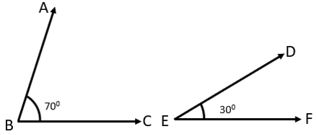Here, ∠ABC + ∠DEF = 70° + 30° = 100°

Sum of both the angles is 100°, hence, ∠ABC and ∠DEF are not complementary.

Example 3. Find the angle which is equal to its complement.

Solution. Let's assume one of the two angles is 'p'.

Hence, p + p = 90°

⇒ 2p = 90°

⇒ p = 90°2

⇒ p = 45°

So, 45° is the angle equal to it's complement.

## Supplementary Angles

Two angles are known as supplementary angles if the sum of their measures is 180°. Each angle is called supplement of the other angle. Let's see some examples to understand it better.

Example 1. Prove that below provided angles are supplementary angles.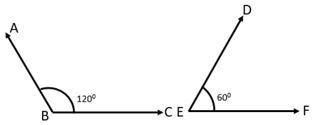Here, ∠ABC + ∠DEF = 120° + 60° = 180°

Sum of both the angles is 180°, hence, ∠ABC and ∠DEF are supplementary.

Example 2. Prove that below provided angles are not supplementary angles.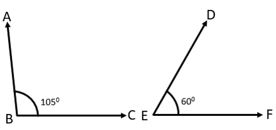Here, ∠ABC + ∠DEF = 105° + 60° = 165°

Sum of both the angles is 165°, hence, ∠ABC and ∠DEF are not supplementary.

Example 3. Find the angle which is equal to it's supplement.

Solution. Let's assume one of the two supplementary angles be 'q'

So, q + q = 180°

⇒ 2q = 180°

⇒ q = 180°2

⇒ q = 90°

Hence, 90° is the angle equal to it's complement.

Two angles are known as adjacent angles if they have a common vertex, a common arm and their non common arms lie on either side of the common arm. Look at the examples given below.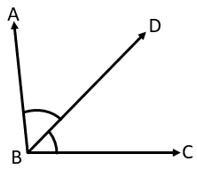Here, ∠ABD and ∠DBC have a common vertex B, common arm is BD, and both the non common arms BA and BC lie on either sides of the common arm BD.

## Linear Pair

Two adjacent angles can form a linear pair if their non common arms are opposite rays as shown in below figure. In other words, if a pair of supplementary angles are placed adjacent to each other, then they will form a linear pair.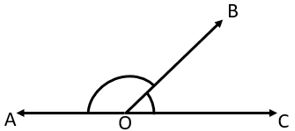⇒ ∠AOC = ∠AOB + ∠BOC

⇒ ∠AOB + ∠BOC = 180°

## Angles About a Point

If a number of angles are formed about a point as shown in the below figure, their sum will always be 360°.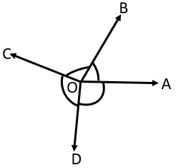∠AOB + ∠BOC + ∠COD + ∠DOA = 360°

Example 1. Find the value of 'x' in the below given diagram.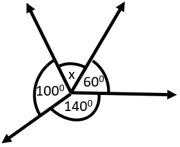Solution. As we know sum of all angles at a point = 360°

⇒ x + 60° + 140° + 100° = 360°

⇒ x + 300° = 360°

⇒ x = 360° − 300°

⇒ x = 60°

Hence, x is 60°.

## Vertically Opposite Angles

When two straight lines intersect each other, they form four angles at the point of intersection. The pair of angles which lie on the opposite sides of the point of intersection are known as vertically opposite angles.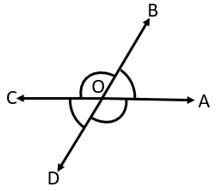∠AOB = ∠COD and ∠BOC = ∠DOA

Example 1. Find the values of p, q, and r in the below diagram.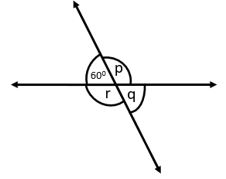Solution. Here, q = 60°

As we know, p + 60° = 180°

⇒ p = 180° − 60°

⇒ p = 120°

p = r = 120°

So, p = 120°, q = 60°, and r = 120°

## Angles on One Side of a Straight Line

Sum of angles at a point on one side of a straight line is equal to 180°.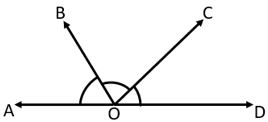∠AOB + ∠BOC + ∠COD = ∠AOD = 180°

Example 1. Find the value of p, q and r in the below diagram.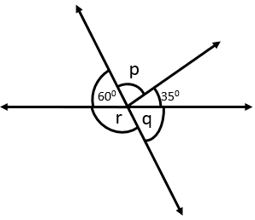Solution. Here, q = 60° (vertically opposite angles)

As the sum of angles at a point on one side of a straight line is 180°.

⇒ 60° + p + 35° = 180°

⇒ p = 180° − 60° − 35°

⇒ p = 85°

⇒ r + q = 180°

⇒ r + 60° = 180°

⇒ r = 180° − 60°

⇒ r = 120°

Hence, p = 85°, q = 60°, and r = 120°.

## Concept of Transversal

A straight line which cuts two or more straight lines is known as transversal.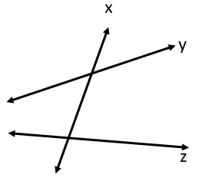In the above figure, line 'x' intersects two lines 'y' and 'z' at different points. Here line 'x' is known as transversal.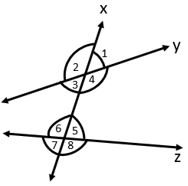Let's discuss different types of angles formed as shown in the above diagram.

## Interior Angles

Here ∠3, ∠4, ∠5 and ∠6 are interior angles.

## Exterior Angles

Here ∠1, ∠2, ∠7 and ∠8 are exterior angles.

## Corresponding Angles

Here four pairs ∠1, ∠5; ∠2, ∠6; ∠3, ∠7; ∠4, ∠8 are called pairs of corresponding angles.

## Alternate Interior Angles

Here ∠3, ∠5 and ∠4, ∠6 are called alternate interior angles.

## Alternate Exterior Angles

Here ∠1, ∠7 and ∠2, ∠8 are called alternate exterior angles.

## Co-interior Angles

Here ∠3, ∠6 and ∠4, ∠5 are called co-interior angles.

## Properties of Angles Made by a Transversal with Two Parallel Lines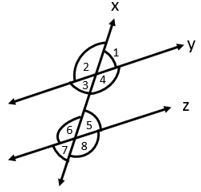Let a traversal 'x' intersect two parallel lines 'y' and 'z' as shown in the above diagram. We can observe that total 8 angles are formed at two intersection point, and the angles are ∠1, ∠2, ∠3, ∠4, ∠5, ∠6, ∠7, ∠8. In the above diagram ∠1 = ∠5 and ∠2 = ∠6, ∠3 = ∠7 and ∠4 = ∠8

Let's see some examples to understand it better.

Example 1. In the below provided diagram y || z. Find the measure of all the marked angles.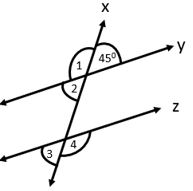Solution. Since 'y' and 'z' are parallel and a transversal 'x' meets them, therefore

∠2 = 45° (vertically opposite angles)

∠2 = ∠3 = 45° (Corresponding angles)

∠1 + 45° = 180° (Linear pair)

⇒ ∠1 = 180° − 45°

⇒ ∠1 = 135°

∠3 + ∠4 = 180°

⇒ 45° + ∠4 = 180° (Linear pair)

⇒ ∠4 = 180° − 45°

⇒ ∠4 = 135°

Example 2. Prove that lines A and B are parallel to each other.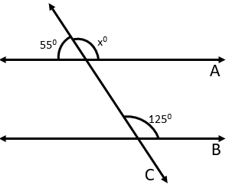Solution. Let's find out angle xo as per linear pair rule.

55° + x° = 180°

⇒ x° = 180° − 55°

⇒ x° = 125°

Here, we can see corresponding angles are equal. Hence it is proved that line A and B are parallel.

Example 3. In the below given diagram AB || CE. Find the value of p, q and r.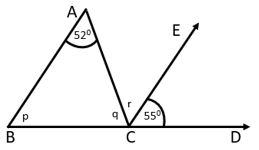Solution. As AB || CE

r = 52° (Alternate angles)

q + r + 55° = 180° (Linear pair)

⇒ q + 52° + 55° = 180°

⇒ q = 180° − 52° − 55°

⇒ q = 73°

Summation of all the angles of a triangle is 180°.

p + q + 52° = 180°

⇒ p + 73° + 52° = 180°

⇒ p = 180° − 73° − 52°

⇒ p = 55°

Hence, p = 55°, q = 73° and r = 52°.

Example 4. In the below given diagram a pair of parallel lines are cut by a transversal. Find the value of 'p'.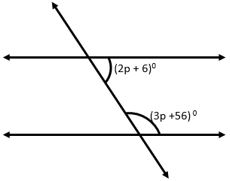Solution. Supplementary angle of (2p+6)° = 180° − (2p+6)°

= 180° − 2p − 6°

= 176° − 2p

As we know, corresponding angles are same

⇒ 176° − 2p = 3p + 56°

⇒ 5p = 176° − 56°

⇒ 5p = 120°

⇒ p = 120°5

⇒ p = 24°

Hence, p is equal to 24°

## Lines and Angles Test

Lines and Angles - 1

Lines and Angles - 2

Lines and Angles - 3

## Lines and Angles Worksheet

Lines and Angles Worksheet - 1

Lines and Angles Worksheet - 2

Lines and Angles Worksheet - 3